Get instant live expert help with Excel or Google Sheets“My Excelchat expert helped me in less than 20 minutes, saving me what would have been 5 hours of work!”

#### Post your problem and you'll get expert help in seconds

Your message must be at least 40 characters
Our professional experts are available now. Your privacy is guaranteed.

# Excel HLOOKUP FunctionRead time: 30 minutes

While working with Excel, we are able to lookup a value from a row across a table and retrieve specific data by using the HLOOKUP function.  HLOOKUP provides a quick way of horizontally looking up a value from any list .  This step by step tutorial will assist all levels of Excel users in the usage and syntax of HLOOKUP function.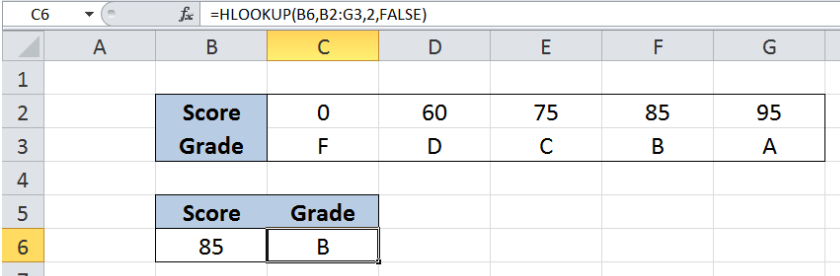Figure 1. Final result: Excel HLOOKUP function

Formula for an exact match:  `=HLOOKUP(B6,B2:G3,2,FALSE)`

Formula for an approximate match: `=HLOOKUP(B6,B2:G3,2,TRUE)`

## Syntax of the HLOOKUP function

HLOOKUP function finds a value in the top row of a data set and returns a value in the same column as the lookup value.  We use HLOOKUP or “Horizontal” Lookup when the comparison values are located at the top row across a table or array containing the data we want to find

`=HLOOKUP(lookup_value, table_array, row_index_num, [range_lookup])`

The parameters of the HLOOKUP function are:

• lookup_value – the value that we want to search and find in the first row of table_array
• table_array – the range of cells in the source table containing the data we want to retrieve
• row_index_num the row number in the table_array corresponding to the information we want to retrieve relative to the lookup_value
• the value 1 refers to the first row of the table array, 2 refers to the second row, and so on
• HLOOKUP returns the #VALUE! error if row_index_num is less than 1, and returns the #REF! error if row_index_num is greater than the number of rows in table_array
• [range_lookup] – optional; value is either TRUE or FALSE
• if TRUE or omitted, HLOOKUP returns either an exact or approximate match
• if FALSE, HLOOKUP will only find an exact match

## Setting up Our Data

Our table consists of two rows: Score (row 2) and Grade (row 3).  In cell B6, we enter the value we want to find which is “85”. We want to look up “85” in the table B2:G3 and return the corresponding grade in cell C6.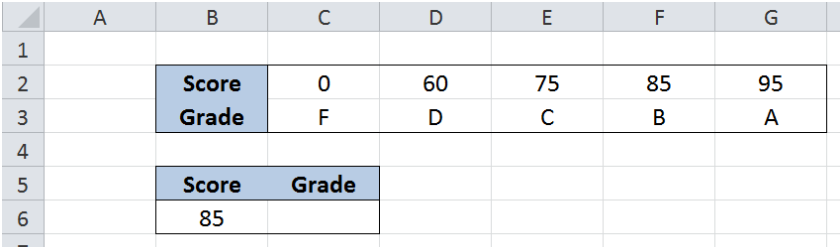Figure 2. Sample data for Excel HLOOKUP function

## Lookup grade using exact match

We want to determine the corresponding grade for the score of “85”.  Let us follow these steps:

Step 1.  Select cell C6

Step 2.  Enter the formula: `=HLOOKUP(B6,B2:G3,2,FALSE)`

Step 3:  Press ENTER

Our lookup_value is cell B6, which contains the specific score we want to search: “85”.  Our table_array is the range B2:G3. “Grade” is in the second row of the table array so row_index_num is 2.  Range_lookup is FALSE because we want to find an exact match.

The final result in cell C6 is B, which is the grade of the score we want to search, “85”.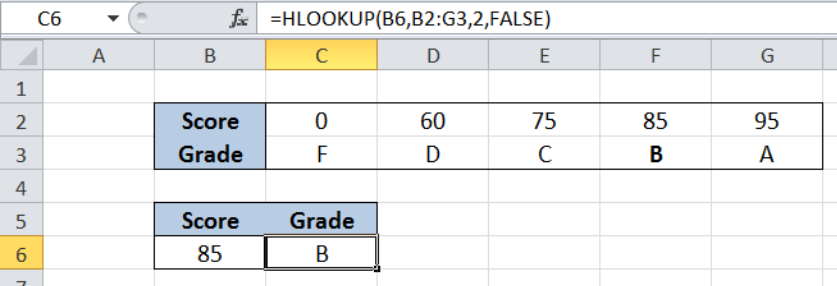Figure 3. Using HLOOKUP and exact match to lookup the grade for score “85”

Now let’s try and search for a different value.  Enter the value “78” in cell B6.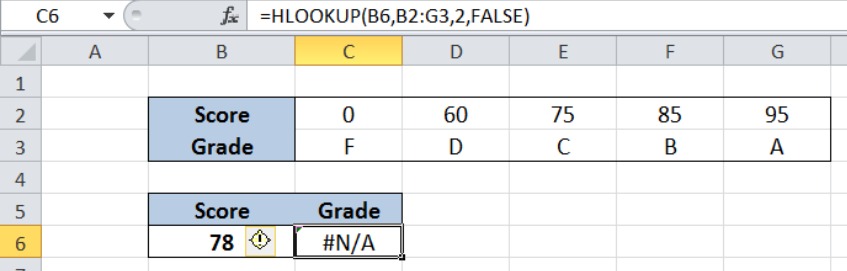Figure 4. HLOOKUP returns an error when lookup value is not found

As shown above, our formula returns the error value #N/A, which means that it wasn’t able to find the lookup value in our table.  By using the range lookup value “FALSE” in our HLOOKUP formula, we are only looking for an exact match. If a value isn’t found, the function returns an error value.

The work-around for cases like this is by using the range lookup value “TRUE”, which returns either an exact or approximate match.

## Lookup grade using approximate match

In order to determine the nearest corresponding grade for a score that is not in the list, we use the HLOOKUP function for an approximate match.  It is important to note that for an approximate match to work properly, the values in the first row must be arranged in ascending order.

Step 1.  Select cell C6

Step 2.  Enter the formula: `=HLOOKUP(B6,B2:G3,2,TRUE)`

Step 3:  Press ENTER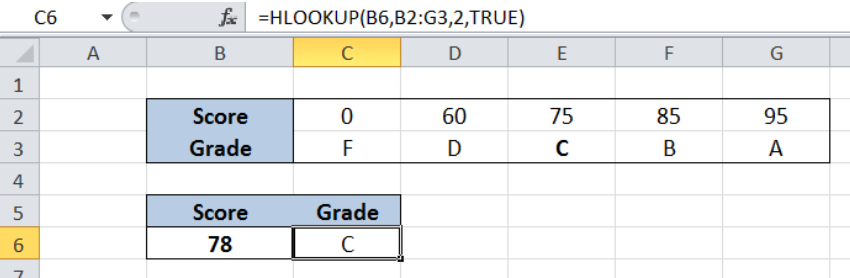Figure 4. HLOOKUP returns grade for closest value less than “78”

The first three arguments of our formula are still the same as in the previous example.  Only the range_lookup value is changed from FALSE to TRUE.

By doing so, our HLOOKUP now searches for the lookup value of “78” and if it is not found, it will consider the closest value that is less than “78”.  In this case, it will identify the score “75” and return the corresponding grade.

The final result in cell C6 is C, which is the grade of score “75”, the closest value less than “78”.

Most of the time, the problem you will need to solve will be more complex than a simple application of a formula or function. If you want to save hours of research and frustration, try our live Excelchat service! Our Excel Experts are available 24/7 to answer any Excel question you may have. We guarantee a connection within 30 seconds and a customized solution within 20 minutes.

### Did this post not answer your question? Get a solution from connecting with the expert.Another blog reader asked this question today on Excelchat:## Subscribe to Excelchat.coAnother blog reader asked this question today on Excelchat: